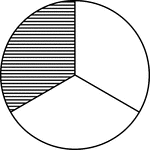### 1/3 Circle

Illustration of a circle divided into thirds. One third is shaded.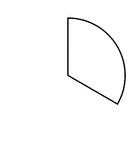### One Third of a Fraction Pie

One third of a circle.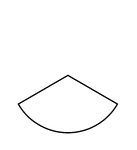### One Third of a Fraction Pie

One third of a circle.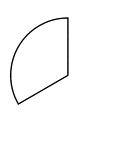### One Third of a Fraction Pie

One third of a circle.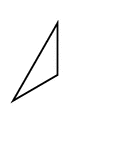### Fractions of 3-sided Polygon

1/3 of a 3 sided polygon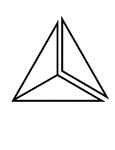### Fractions of 3-sided Polygon

3/3 of a 3 sided polygon with one piece detached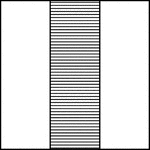### 1/3 Square

Illustration of a square divided into thirds. One third is shaded.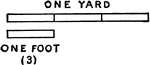### Yard And Foot

Illustration showing that one foot is one third of a yard.